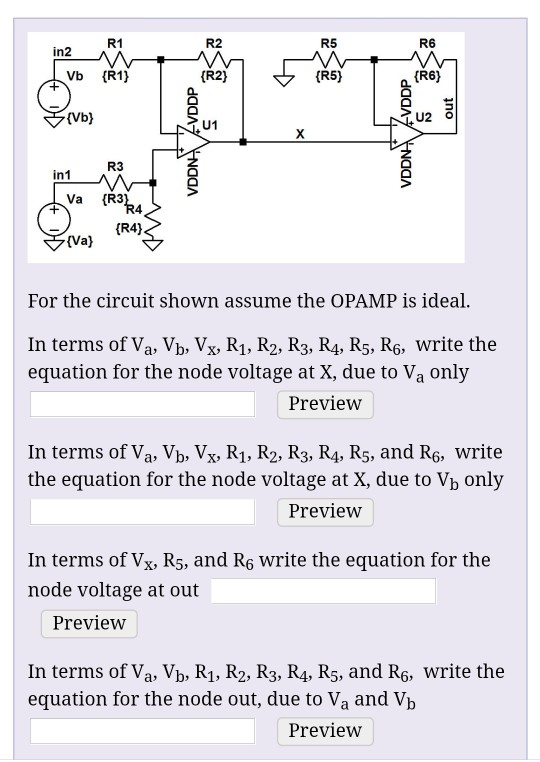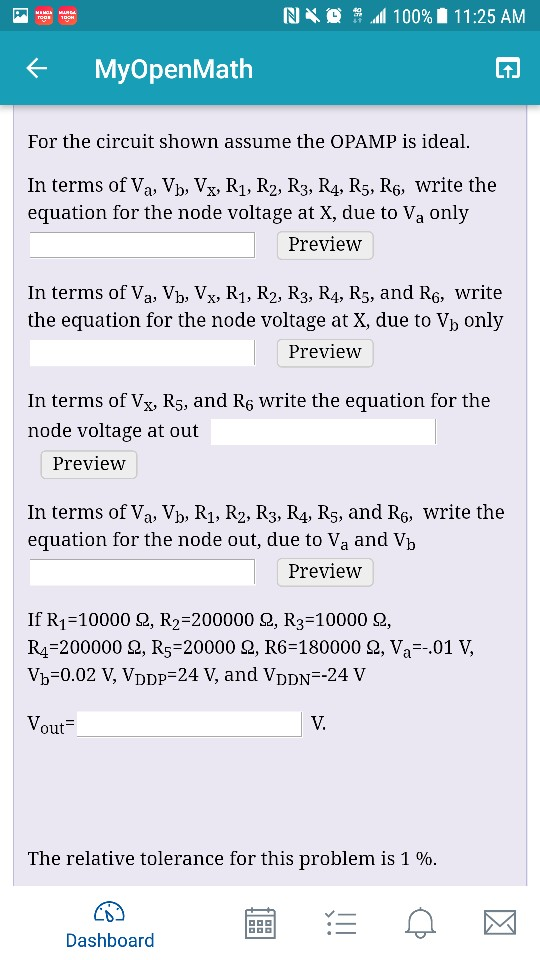# Question R1 R2 R5 R6 in2 Vb (R1) (R2) (R5) (R6) (Vb) U1 U2 O R3 in1 Va (R3 4 (R4) (Va) For the circuit shown assume the OPAMP is ideal In terms of Va, Vb, Vx, R1, R2, R3, R4, R5, R6, write the equation for the node voltage at X, due to Va only Preview In terms of Va, Vb, Vx, R1, R2, R3, R4, R5, and R6, write the equation for the node voltage at X, due to Vb only R1 R2 R5 R6 in2 Vb (R1) (R2) (R5) (R6) (Vb) U1 U2 O R3 in1 Va (R3 4 (R4) (Va) For the circuit shown assume the OPAMP is ideal In terms of Va, Vb, Vx, R1, R2, R3, R4, R5, R6, write the equation for the node voltage at X, due to Va only Preview In terms of Va, Vb, Vx, R1, R2, R3, R4, R5, and R6, write the equation for the node voltage at X, due to Vb only Preview In terms of Vx, R5, and R6 Write the equation for the node voltage at out Preview In terms of Va, Vb, R1, R2, R3, R4, R5, and Rç, write the equation for the node out, due to Va and Vb PreviewZSTLP5 The Asker · Electrical EngineeringTranscribed Image Text: R1 R2 R5 R6 in2 Vb (R1) (R2) (R5) (R6) (Vb) U1 U2 O R3 in1 Va (R3 4 (R4) (Va) For the circuit shown assume the OPAMP is ideal In terms of Va, Vb, Vx, R1, R2, R3, R4, R5, R6, write the equation for the node voltage at X, due to Va only Preview In terms of Va, Vb, Vx, R1, R2, R3, R4, R5, and R6, write the equation for the node voltage at X, due to Vb only Preview In terms of Vx, R5, and R6 Write the equation for the node voltage at out Preview In terms of Va, Vb, R1, R2, R3, R4, R5, and Rç, write the equation for the node out, due to Va and Vb Preview
More
Transcribed Image Text: R1 R2 R5 R6 in2 Vb (R1) (R2) (R5) (R6) (Vb) U1 U2 O R3 in1 Va (R3 4 (R4) (Va) For the circuit shown assume the OPAMP is ideal In terms of Va, Vb, Vx, R1, R2, R3, R4, R5, R6, write the equation for the node voltage at X, due to Va only Preview In terms of Va, Vb, Vx, R1, R2, R3, R4, R5, and R6, write the equation for the node voltage at X, due to Vb only Preview In terms of Vx, R5, and R6 Write the equation for the node voltage at out Preview In terms of Va, Vb, R1, R2, R3, R4, R5, and Rç, write the equation for the node out, due to Va and Vb Preview